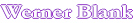Home

VISCOSITY SOLIDS RELATIONSHIP OF A POLYMER SOLUTION USING TWO DATA POINTS

WLF equation

This program assumes that the glass transition temperature  (Tg)of a polymer decreases linearly with the weight fraction of solvent. Using the WLF equation (William, Landel and Ferry Equation) the viscosity of the polymer solution can be calculated from the Tg. Universal constants according to Nielsen are used for this calculation. This program also assumes that no unusual interaction polymer-solvent is taking place. The largest error introduced into this calculation comes from the solids determination.
 Log η= C-A(T-Tg)/(B+(T-Tg)) C= Universal Constants for most polymers A= B=
Tgs = Co +C1Ws Tgs = solution Tg Co, C1= constants for polymer solution Ws = weight fraction of solvent
The constants Co, C1 are specific for a polymer and a solvent or solvent blend. Co corresponds to the Tg of the polymer expressed in ¯K
Measured Data Viscosity, cps Weight, % Temp. ¤C Poise  Visc. log T-Tg Tg
DATA POINT 1
DATA POINT 2

Calculated Constants

Co =

C1 =

Enter desired data
Weight, %
Delta, %
Temperature, ¤C
Delta, ¤C
Calculated Data Temperature, ¤C

Weight, %

Ws Tgs

Viscosity, cps

M.L.Williams, R.F.Landel and  J.D.Ferry, J.Am.Chem.Soc.77,3701(1955)

L.E.Nielsen, Polymer Rheology, Marcel Dekker, 1977,pp.33,74,133

Automatic recalculation

 Last edited on: October 20, 2010 CopyrightÛ, Design, Layout and Technical Content by: